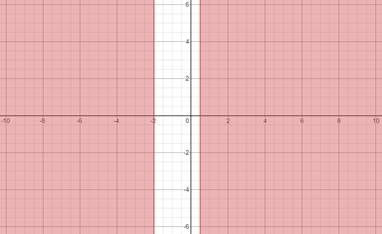# Find the solution of the equation using graph.### Precalculus: Mathematics for Calcu...

6th Edition
Stewart + 5 others
Publisher: Cengage Learning
ISBN: 9780840068071### Precalculus: Mathematics for Calcu...

6th Edition
Stewart + 5 others
Publisher: Cengage Learning
ISBN: 9780840068071

#### Solutions

Chapter 1.9, Problem 68E
To determine

## Find the solution of the equation using graph.

Expert Solution

x(,2][12,+)

### Explanation of Solution

Given information:

5x2+3x3x2+2

The graph for equation 5x2+3x3x2+2The solution of the equation 5x2+3x3x2+2 is x(,2][12,+)

### Have a homework question?

Subscribe to bartleby learn! Ask subject matter experts 30 homework questions each month. Plus, you’ll have access to millions of step-by-step textbook answers!